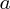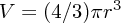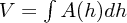## Volume

• The volume of an object is a three-dimensional measurement of the space it occupies. The formulae for the volumeof common shapes are:

 shape formula note cubewhereis the length of the sides spherewhereis the radius cylinderwhereis the radius andis the height generalwhereis the height andis the area of a cross-section perpendicular toas a function of